# AVL Tree | AVL Tree Example | AVL Tree Rotation

## AVL Tree-

• AVL trees are special kind of binary search trees.
• In AVL trees, height of left subtree and right subtree of every node differs by at most one.
• AVL trees are also called as self-balancing binary search trees.

## Example-

Following tree is an example of AVL tree-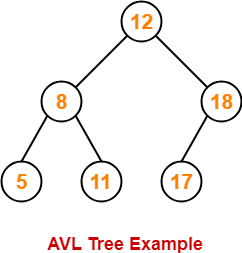This tree is an AVL tree because-

• It is a binary search tree.
• The difference between height of left subtree and right subtree of every node is at most one.

Following tree is not an example of AVL Tree-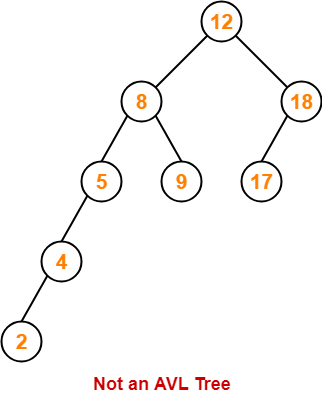This tree is not an AVL tree because-

• The difference between height of left subtree and right subtree of root node = 4 – 2 = 2.
• This difference is greater than one.

## Balance Factor-

In AVL tree,

• Balance factor is defined for every node.
• Balance factor of a node = Height of its left subtree – Height of its right subtree

 In AVL tree, Balance factor of every node is either 0 or 1 or -1.

## AVL Tree Operations-

Like BST Operations, commonly performed operations on AVL tree are-

1. Search Operation
2. Insertion Operation
3. Deletion Operation

Also Read- Insertion in AVL Tree

After performing any operation on AVL tree, the balance factor of each node is checked.

There are following two cases possible-

### Case-01:

• After the operation, the balance factor of each node is either 0 or 1 or -1.
• In this case, the AVL tree is considered to be balanced.
• The operation is concluded.

### Case-02:

• After the operation, the balance factor of at least one node is not 0 or 1 or -1.
• In this case, the AVL tree is considered to be imbalanced.
• Rotations are then performed to balance the tree.

## AVL Tree Rotations-

 Rotation is the process of moving the nodes to make tree balanced.

## Kinds of Rotations-

There are 4 kinds of rotations possible in AVL Trees-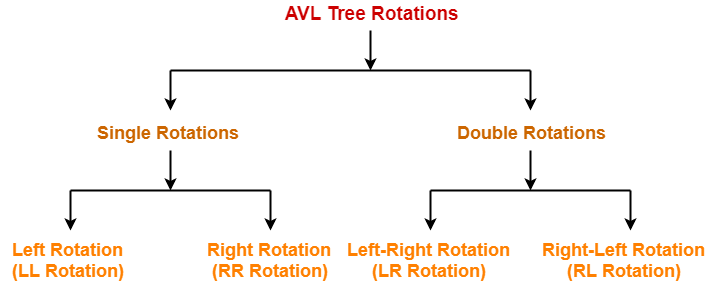1. Left Rotation (LL Rotation)
2. Right Rotation (RR Rotation)
3. Left-Right Rotation (LR Rotation)
4. Right-Left Rotation (RL Rotation)

### Case-01: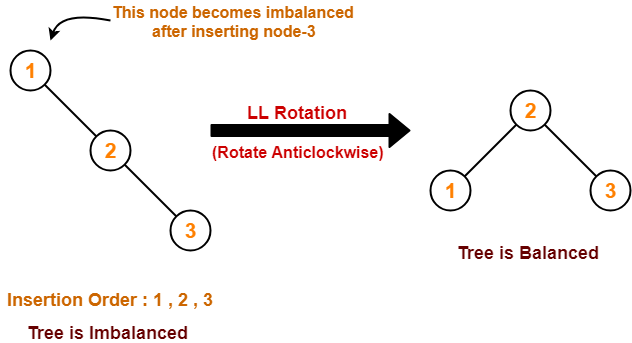### Case-02: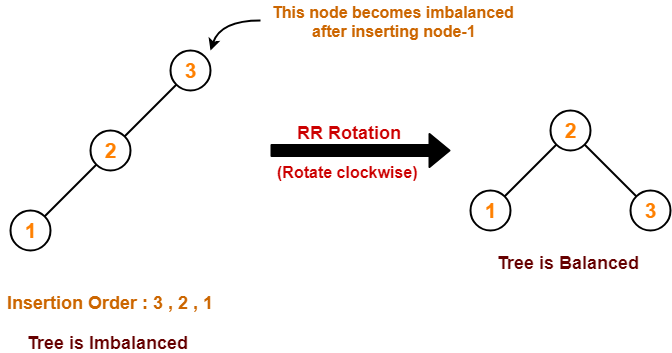### Case-03: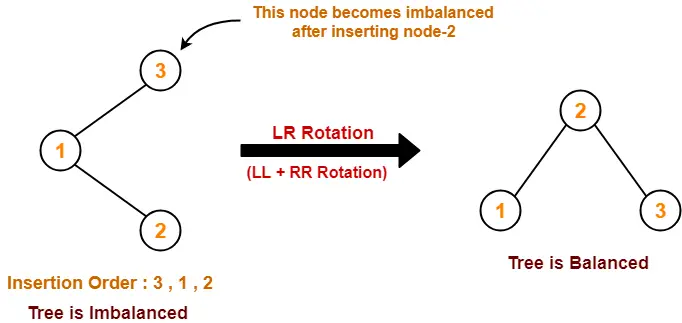### Case-04: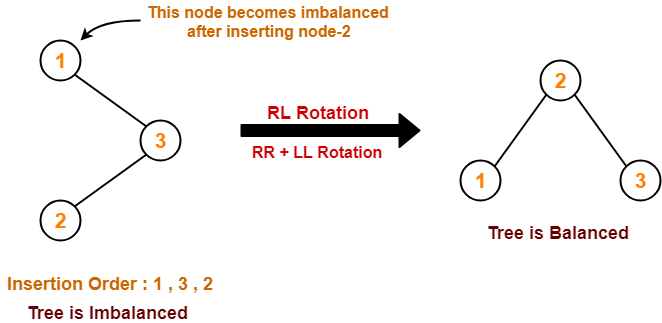To gain better understanding about AVL Trees and Rotations,

Watch this Video LectureNext Article- AVL Tree Properties

Get more notes and other study material of Data Structures.

Watch video lectures by visiting our YouTube channel LearnVidFun.

SummaryArticle Name
AVL Tree | AVL Tree Example | AVL Tree Rotation
Description
AVL Tree in data structure is a self balancing binary search tree. AVL Tree Examples are given. AVL Tree Rotations refer to the process of moving nodes to make the tree balanced.
Author
Publisher Name
Gate Vidyalay
Publisher Logo